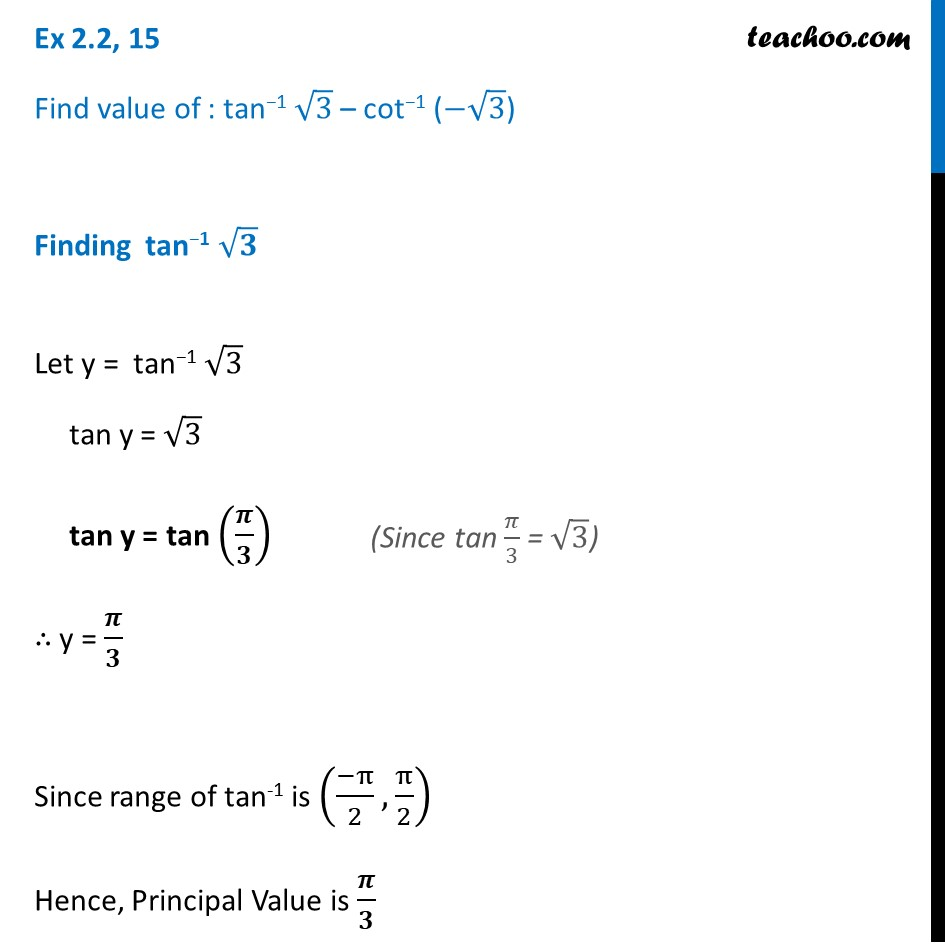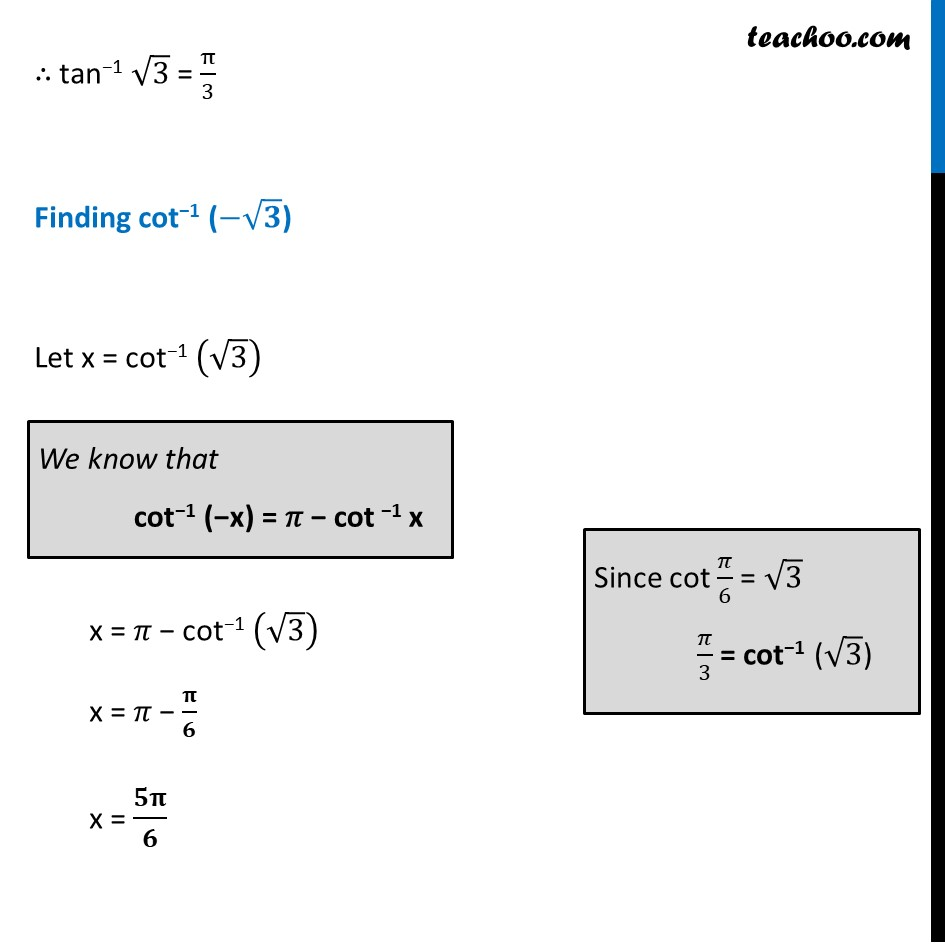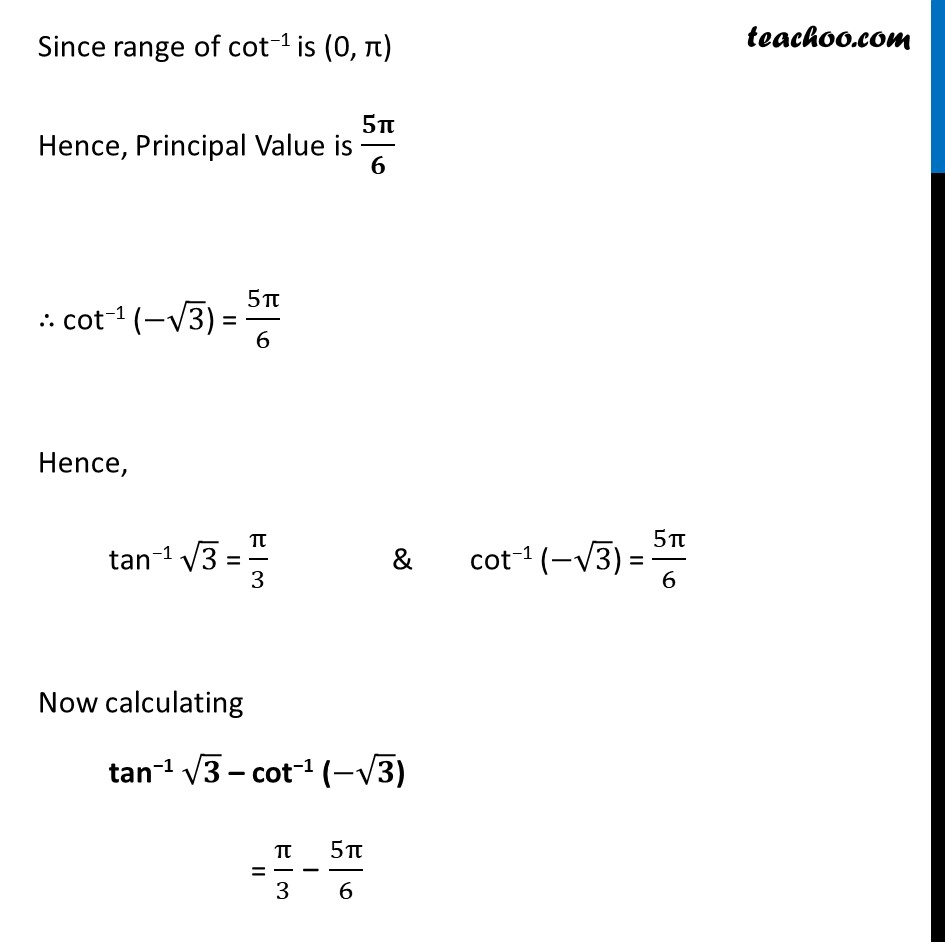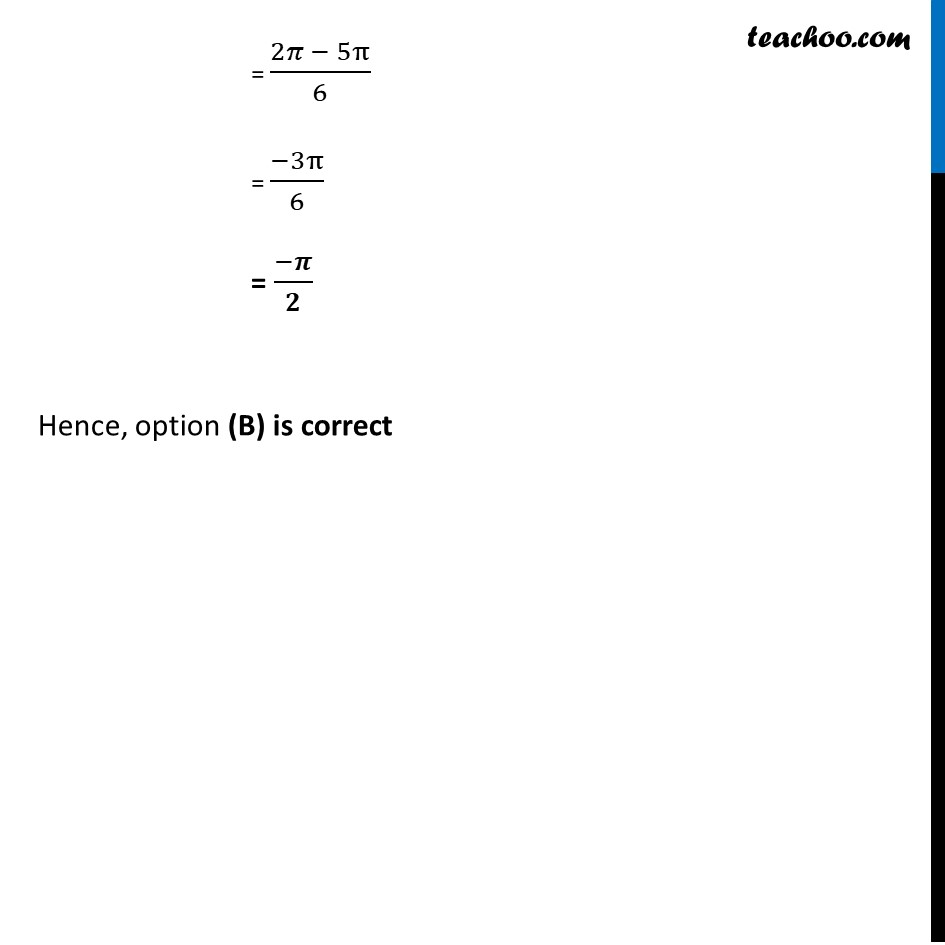Ex 2.2

Chapter 2 Class 12 Inverse Trigonometric Functions
Serial order wiseLearn in your speed, with individual attention - Teachoo Maths 1-on-1 Class

### Transcript

Ex 2.2, 15 Find value of : tan−1 √3 – cot−1 (−√3) Finding tan−1 √𝟑 Let y = tan−1 √3 tan y = √3 tan y = tan (𝝅/𝟑) ∴ y = 𝝅/𝟑 Since range of tan-1 is ((−π)/2,π/2) Hence, Principal Value is 𝝅/𝟑 ∴ tan−1 √3 = π/3 Finding cot−1 (−√𝟑) Let x = cot−1 (√3) x = 𝜋 − cot−1 (√3) x = 𝜋 − 𝛑/𝟔 x = 𝟓𝛑/𝟔 We know that cot−1 (−x) = 𝜋 − cot −1 x Since cot 𝜋/6 = √3 𝜋/3 = cot−1 (√3) Since range of cot−1 is (0, π) Hence, Principal Value is 𝟓𝛑/𝟔 ∴ cot−1 (−√3) = 5π/6 Hence, tan−1 √3 = π/3 & cot−1 (−√3) = 5π/6 Now calculating tan−1 √𝟑 – cot−1 (−√𝟑) = π/3 − 5π/6 = (2𝜋 − 5π)/6 = (−3π)/6 = (−𝝅)/𝟐 Hence, option (B) is correct